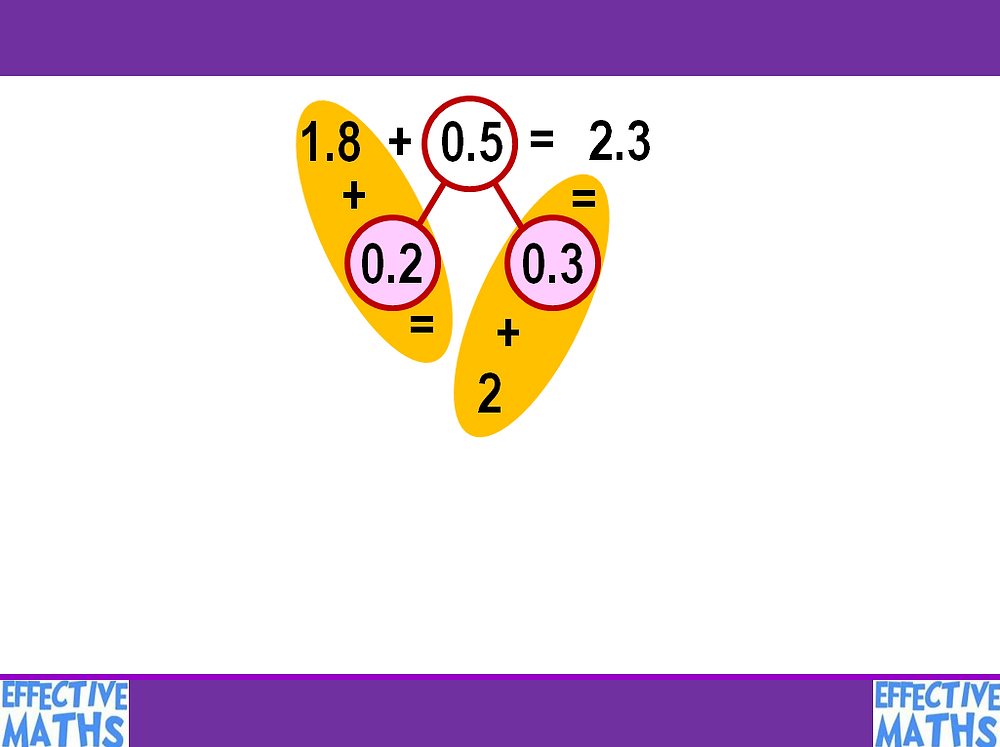Search
•Effective Maths

# WEEK 4 OF THE EFFECTIVE MATHS ARITHMETIC AND NUMBER FACTS REVISION PROGRAMME IS NOW ONLINE

WEEK 4 OF THE EFFECTIVE MATHS ARITHMETIC AND NUMBER FACTS REVISION PROGRAMME IS NOW ONLINE

The focuses for week 4 are:

4F8 compare numbers with the same number of decimal places up to two decimal places

5F8 read, write, order and compare numbers with up to three decimal places

5F10 solve problems involving numbers up to three decimal places

6F9a identify the value of each digit to three decimal places and multiply and divide numbers by 10, 100 and 1,000 giving answers up to three decimal places

6F9b multiply one-digit numbers with up to two decimal places by whole numbers